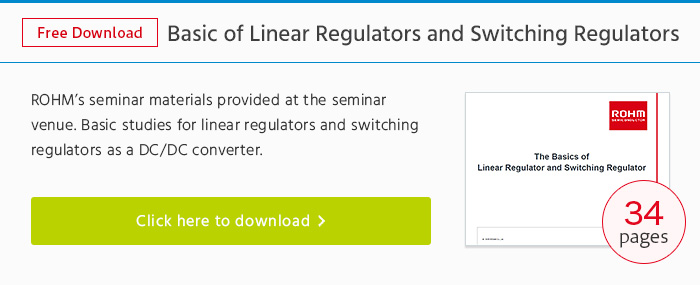# Variable voltage and Fixed voltage＜Operating Principle of Linear Regulators＞

## General Pin Configuration

Linear regulators basically consist of 3 terminals, VIN (input), VO (output), and GND (ground).

For those types where the output can be varied, a feedback terminal FB is added to feedback the output voltage.

In short, a fixed voltage type is a variable voltage type that integrates the external resistor.## Internal Circuit

An overview of the internal circuit of a linear regulator is shown in the figure below.It basically consists of an error amp for error detection, reference voltage source, and output transistor.

Since operation is the same as that of an inverting amplifier circuit, the voltage at the non-inverting terminal (FB) of the error amp will become the same as the reference voltage (VREF), with the output voltage (VO) determined by the resistance value ratio (R1 and R2).

Vo=[ (R1 + R2) / R2 ] × VREF

The output transistor shown at right is a MOSFET. However, bipolar transistors are also used.